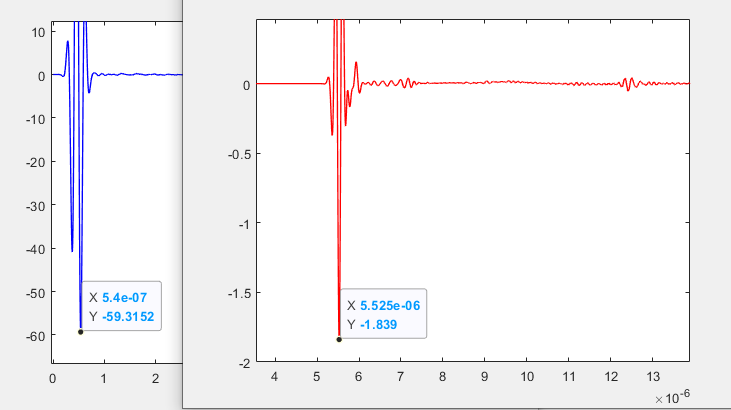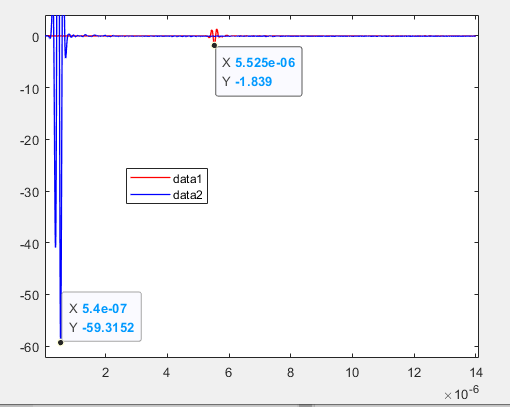# The velocity of longitudinal wave changes

Like the following code，I set the velocity of L-wave of the material of my model  to 5900m/s.

`c Materialsmatr	type elas	wvsp on	prop steel 7900. 5900. 3200.		/* Create tx and rx elements	do loopI I 1 \$ele_numx 1	do loopJ J 1 \$ele_numy 1	prop etx\$I/\$J 0. 0. 0.	end\$ loopJ	end\$ loopI	endc Assign materials to gridsite	/* Make all elements void	regn void		/* Create plate	regn steel \$i1 \$indgrd \$j1 \$jndgrd \$k1 \$k3		/* Create tx array elements	do loopI I 1 \$ele_numx 1		symb iend = 2 * \$I + 1 		symb ibeg = \$iend - 1		do loopJ J 1 \$ele_numy 1		symb jend = 2 * \$J + 1		symb jbeg = \$jend - 1		regn etx\$I/\$J \$i\$ibeg \$i\$iend \$j\$jbeg \$j\$jend \$k3 \$k4		end\$ loopJ	end\$ loopI		/* Defect	sphr void \$def_x \$def_y \$def_z \$def_rad	end`

Then I extract the aprs of tow points along the path and direction of L-wave.The distance of  them is 29.5mm.

`poutc form matlab h1 /* uncomment if Matlab output format is desiredhist funchistname avrg * allhist xyz aprs 1.8e-3 1.8e-3 1 2.4e-3 2.4e-3 1 10e-3 10e-3 1hist xyz aprs 1.8e-3 1.8e-3 1 2.4e-3 2.4e-3 1 39.5e-3 39.5e-3 1end`

Then I plot the A scan signal of the two points in time domain using Matlab and extract the corresponding time of two points.The velocity of the wave from the first point to the second point is calculated as 5917.8m/s approximately.Why?How to calibrate？